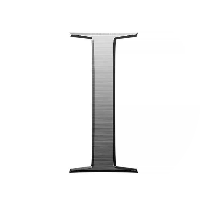# Groups: Axioms, Theories and Properties

This quiz contains multiple-choice problems on group theory and axioms, closure and associativity, subgroups, identity and inverse existence, Burnside’s theorem, cyclic and permutation groups.

Start Quiz

Let (A7, ⊗7) = ({1, 2, 3, 4, 5, 6}, ⊗7) is a group. It has two subgroups, X and Y, where X={1, 3, 6}, Y={2, 3, 5}. What is the order of the union of subgroups?

65

5

32

18

B1: ({0, 1, 2….(n-1)}, xm), where xn stands for “multiplication-modulo-n”

B2: ({0, 1, 2….n}, xn), where xn stands for “multiplication-modulo-m”

Both B1 and B2 are considered to be

Groups

Semigroups

Subgroups

Associative subgroups

If group G has 65 elements and two subgroups, namely K and L, with orders 14 and 30, respectively, what can be the order of K intersection L?

10

42

5

35

Consider the binary operations on X for a, b ∈ X, a*b = a + b + 4. It satisfies the properties of

Abelian group

Semigroup

Multiplicative group

Isomorphic group

Let * be the binary operation on the rational number given by a*b = a + b + ab. Which of the following properties does not exist for the group?

Closure property

Identity property

Symmetric property

Associative property

Let G be a finite group with two subgroups, M and N, such that |M| = 56 and |N| = 123. Determine the value of |M⋂N|.

1

56

14

78

A group G, ({0}, +) under addition operation satisfies which of the following properties?

Identity, multiplicity and inverse

Closure, associativity, inverse and identity

Multiplicity, associativity and closure

Inverse and closure

If (M, *) is a cyclic group of order 73, then the number of generators of G is equal to

89

23

72

17

The set of even natural numbers, {6, 8, 10, 12,..,} is closed under addition operation. Which of the following properties will it satisfy?

Closure property

Associative property

Symmetric property

Identity property

A trivial subgroup consists of

Identity element

Coset

Inverse element

Ring

The minimum subgroup of a group is called a

Commutative subgroup

Lattice

Trivial group

Monoid

Let K be a group with eight elements. Let H be a subgroup of K and H < K. It is known that the size of H is at least 3. The size of H is

8

2

3

4

__ is not necessarily a group property

Commutativity

Existence of inverse for every element

Existence of Identity

Associativity

A group of rational numbers is an example of a

Subgroup of a group of integers

Subgroup of a group of real numbers

Subgroup of a group of irrational numbers

Subgroup of a group of complex numbers

A relation (34 × 78) × 57 = 57 × (78 × 34) can have the __ property.

Distributive

Associative

Commutative

Closure

Quiz/Test Summary
Title: Groups: Axioms, Theories and Properties
Questions: 15
Contributed by: